# GeometryData Sufficiency Videos

Every Data Sufficiency (DS) question can have 1 of 5 possible answers, and the possible answers are the same for every DS question. All DS questions are really two questions in one. You’re given a general problem, followed by two supplemental statements. The real problem is to evaluate each of the two statements independently to determine their individual sufficiency to solve the original general problem. As you determine the sufficiency of these statements, you are able to decide which of the following 5 answers to the Data Sufficiency question is the correct answer:

A. Statement 1 is sufficient, but statement 2 is not.

B. Statement 1 is not sufficient, but statement 2 is.

C. Neither statement 1 nor statement 2 is sufficient, but together they are.

D. Both statement 1 and statement 2 are individually sufficient.

E. Neither statement 1 nor statement 2 is sufficient, and together they are not sufficient.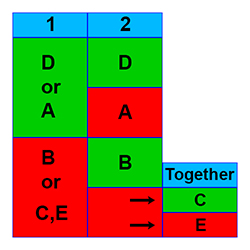The diagram above gives a pictorial representation of the 5 Data Sufficiency answers. The two statement numbers are shown in blue at the top of the first two columns. Note that green means sufficient, and red means not sufficient. As an example, if both statements 1 and 2 are individually sufficient, then the correct answer is D. For more information on the 5 DS answers, see our videos.

We illustrate these answers for geometry problems with the following 8 examples. Each of the first two examples provides 5 different versions of supplementary statements for the same general problem, and each of these 5 versions yields a different one of the 5 possible answers. The answers to these 5 versions are explained immediately after the problem. After the first 2 examples, we then give 6 additional examples with just one version of the supplementary statements.

[Notice in the first 2 examples that the 5 versions of the supplementary statements provide examples of the 5 different answers, A – E. However, they are not given in A – E order.]

Example 1)

A triangle, XYZ, is inscribed in a circle with one of its sides being a diameter of the circle. What is the area of the triangle?

Version I.

(1) The area of the circle is 25π.
(2) The length of one of the sides of the triangle other than the diameter is 8.

Version II.

(1) The area of the circle is 25π.
(2) The circumference of the circle is 10π.

Version III.

(1) The circumference of the circle is 10π.
(2) The area of the circle is 25π, and the length of one of the other sides of the triangle is 6.

Version IV.

(1) The area of the circle is 25π, and the length of one of the other sides of the triangle is 6.
(2) The circumference of the circle is 10π, and the area of the part of the circle outside the triangle is 25π – 24.

Version V.

(1) The circumference of the circle is 10π, and the area of the part of the circle outside the triangle is 25π – 24.
(2) The length of one of the sides of the triangle other than the diameter is 8.

Answers: [ Version I: C Version II: E Version III: B Version IV: D Version V: A ]

Explanation:

If a triangle is inscribed in a circle, and one of its sides is a diameter, then that triangle is a right triangle, and the diameter is the hypotenuse. Thus, if we know the diameter of the circle, and the length of one other side of the triangle, we can use the Pythagorean Theorem to determine the length of the third side. The area of the triangle is one-half the product of the lengths of the two sides other than the hypotenuse.

Version I: Answer C – Neither statement 1 nor statement 2 is sufficient, but together they are.(1) The area of the circle is 25π.

Partial information. Knowing the area of the circle we can easily compute the radius, which we get from the formula \begin{align}A = πr^2.\end{align} In this case, the radius is 5. The diameter is just twice the radius (10), and the diameter is the hypotenuse of the triangle. But without the length of one of the other sides of the triangle we can’t go any further. Not sufficient.

(2) The length of one of the sides of the triangle other than the diameter is 8.

Partial information. This gives us the length of one side of the triangle. To compute the area, we need the length of at least one more side. Not sufficient.

Alone, neither of these statements is sufficient, but together they are sufficient, because they provide the lengths of two of the sides of the right triangle, and therefore we can calculate the length of the third side and also the area.

Version II: Answer E – Neither statement 1 nor statement 2 is sufficient, and together they are not sufficient.(1) The area of the circle is 25π.

Partial information. Knowing the area of the circle we can calculate the radius and diameter, which gives us the hypotenuse of the triangle (10). But we need to know the length of one of the other sides to get the third side. Not sufficient.

(2) The circumference of the circle is 10π.

Partial information. Knowing the circumference of the circle we can calculate the diameter, which gives us the hypotenuse of the triangle (10). But we need to know the length of one of the other sides to get the third side. Not sufficient.

Alone, neither of these statements is sufficient, and together they are not sufficient, because they only give us the diameter of the circle, and we need to know the length of at least one other side of the triangle.

Version III: Answer B – Statement 1 is not sufficient, but statement 2 is.(1) The circumference of the circle is 10π.

Partial information. Knowing the circumference of the circle we can calculate the diameter, which gives us the hypotenuse of the triangle (10). But we need to know the length of one of the other sides to get the third side. Not sufficient.

(2) The area of the circle is 25π, and the length of one of the other sides of the triangle is 6.

Full information. Knowing the area of the circle we can easily compute the radius, which we get from the formula \begin{align}A = πr^2.\end{align} In this case, the radius is 5. The diameter is just twice the radius (10), and the diameter is the hypotenuse of the triangle. Knowing the length of one of the other sides of the triangle is 6, we can calculate the third side as 8. Now we can calculate the area of the triangle. Sufficient.

Version IV: Answer D – Both statement 1 and statement 2 are individually sufficient.(1) The area of the circle is 25π, and the length of one of the other sides of the triangle is 6.

Full information. Knowing the area of the circle we can easily compute the radius, which we get from the formula \begin{align}A = πr^2.\end{align} In this case, the radius is 5. The diameter is just twice the radius (10), and the diameter is the hypotenuse of the triangle. Knowing the length of one of the other sides of the triangle is 6, we can calculate the third side as 8. Now we can calculate the area of the triangle. Sufficient.

(2) The circumference of the circle is 10π, and the area of the part of the circle outside the triangle is 25π – 24.

Full information. From the first half of this statement we deduce that the radius of the circle is 5, so its area is 25π. From this and the second half of the statement we deduce that the area of the triangle must be 24. Sufficient.

Version V: Answer A – Statement 1 is sufficient, but statement 2 is not.(1) The circumference of the circle is 10π, and the area of the part of the circle outside the triangle is 25π – 24.

Full information. From the first half of this statement we deduce that the radius of the circle is 5, so its area is 25π. From this and the second half of the statement we deduce that the area of the triangle must be 24. Sufficient.

(2) The length of one of the sides of the triangle other than the diameter is 8.

Partial information. This gives us the length of one side of the triangle. To compute the area, we need the length of at least one more side. Not sufficient.

Example 2)

A rope hanging vertically has 7 equally spaced knots, which we label from bottom to top as A, B, C, D, E, F, and G. Is the distance from knot F to knot G greater than or equal to 18 inches?

Version I.

(1) The distance from A to D is not less than 60 inches.
(2) The distance from D to F is over 30 inches.

Version II.

(1) The distance from A to D is not less than 60 inches.
(2) The distance from A to G is not less than 108 inches.

Version III.

(1) An eighth knot, H, above G, is 30 inches from F.
(2) The distance from F to an eighth knot, H, above G, is 3/2 the distance from F to G.

Version IV.

(1) The distance from D to F is over 30 inches.
(2) The distance from A to E is twice the distance from E to G.

Version V.

(1) The distance from A to E is twice the distance from E to G.
(2) The distance from A to G is not less than 108 inches.

Answers: [ Version I: A Version II: D Version III: C Version IV: E Version V: B ]

Explanation:

These 7 knots are equally spaced, so the distance between any two consecutive knots is the same, regardless of the two knots selected. This is important, because even though we need the distance between F and G, we can find it by knowing the distance between B and C, or D and E, or any other consecutive pair.

Version I: Answer A – Statement 1 is sufficient, but statement 2 is not.(1) The distance from A to D is not less than 60 inches.

Full information. From A to D is 3 knots. If this is not less than 60 inches, then the distance from A to B, from B to C, and from C to D, is not less than 20 inches each. This answers the question. Sufficient.

(2) The distance from D to F is over 30 inches.

Partial information. This is inconclusive, because it merely says the distance is over 30 inches, but it doesn’t say how much over. The distance could easily be 36 or 40 inches, because both of those are over 30. On the other hand, the distance could also be 32 inches, in which case the separation from each pair of knots would be 16 inches. Not sufficient.

Version II: Answer D – Both statement 1 and statement 2 are individually sufficient.(1) The distance from A to D is not less than 60 inches.

Full information. From A to D is 3 knots. If this is not less than 60 inches, then the distance from A to B, from B to C, and from C to D, is not less than 20 inches each. This answers the question. Sufficient.

(2) The distance from A to G is not less than 108 inches.

Full information. This is the full distance from the first knot to G. Or in other words, the distance between knot A and six other knots. If we divide 108 by 6, we get 18, which shows the distance between any two knots must be at least 18 inches. Sufficient.

Version III: Answer C – Neither statement 1 nor statement 2 is sufficient, but together they are.(1) An eighth knot, H, above G, is 30 inches from F.

Partial information. Since we don’t know how far H is from G, we can’t tell from this what the distance is from F to G. Not sufficient.

(2) The distance from F to an eighth knot, H, above G, is 3/2 the distance from F to G.

Partial information. This gives us only a relationship between distances, and we need an absolute number. Not sufficient.

Alone, neither of these statements is sufficient, but together they are sufficient, because if both are true, then the distance from F to G must be 20 inches.

Version IV: Answer E – Neither statement 1 nor statement 2 is sufficient, and together they are not sufficient.(1) The distance from D to F is over 30 inches.

Partial information. This is inconclusive, because it merely says the distance is over 30 inches, but it doesn’t say how much over. The distance could easily be 36 or 40 inches, because both of those are over 30. On the other hand, the distance could also be 32 inches, in which case the separation from each pair of knots would be 16 inches. Not sufficient.

(2) The distance from A to E is twice the distance from E to G.

No information. This states merely that 4 knot-to-knot lengths are twice 2 knot-to-knot lengths, which we knew already. Not sufficient.

Alone, neither of these statements is sufficient, and together they are not sufficient, because statement 1 is inconclusive, and statement 2 gives us no new information.

Version V: Answer B – Statement 1 is not sufficient, but statement 2 is.(1) The distance from A to E is twice the distance from E to G.

No information. This states merely that 4 knot-to-knot lengths are twice 2 knot-to-knot lengths, which we knew already. Not sufficient.

(2) The distance from A to G is not less than 108 inches.

Full information. This is the full distance from the first knot to the last. Or in other words, the distance between knot A and six other knots. If we divide 108 by 6, we get 18, which shows the distance between any two knots must be at least 18 inches. Sufficient.

Example 3)

How many distinct lines on the plane can be created from a set of x points, if y and only y of the points are collinear – that is, all of these y points lie on a single line – and no other set of 3 or more points in the set are collinear?

(1) x < 11
(2) y = 7 and x – y = 3

Answer: B – Statement 1 is not sufficient, but statement 2 is.

Explanation:

Two points determine a line, so ordinarily x points would determine the number of lines arising from the combinations of x objects taken 2 at a time. However, if some of the points are collinear, then this greatly reduces the number of lines determined. Suppose, for example, we have 4 points, and no 3 are collinear. Then these would normally determine 6 lines. But if 3 are collinear, then the 1 non-collinear point will determine 3 lines – 1 line to each of the 3 collinear points – and the other 3 points would determine just 1 line. Altogether there would be 4 lines, instead of 6. In general, if we know the number of points, x, and the number of collinear points, y, we have sufficient information to calculate the number of lines.(1) x < 11

Partial information. This sets an upper bound for x, but without knowing y we can’t figure the number of lines. Not sufficient.

(2) y = 7 and x – y = 3

Full information. 7 points are collinear, and the number of points is 10. Sufficient.

Example 4)

A large inverted cone, 6 feet in height, is partially filled with water. What is the volume of water in the cone? [Note: the volume of a cone is \begin{align}1/3πr^2h\end{align}, where r is the radius of the circle at the base, and h is the height of the cone. If the cone is inverted, then the base is at the top of the cone.]

(1) The diameter of the circle at the top of the cone is 4.
(2) The circle at the top of the cone has an area 4 times that of the circle made by the surface of the water.

Answer: C – Neither statement 1 nor statement 2 is sufficient, but together they are.

Explanation:

The water in the cone makes a cone itself. To calculate its volume you only need to know the height of this water cone and the radius of the circle made by its surface.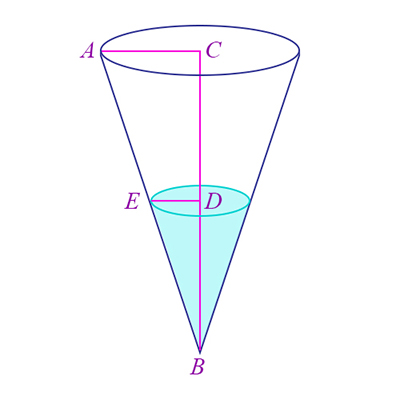A cone has a circular base, and the area of the circle is \begin{align}πr^2,\end{align} where r (CA in the diagram) is the radius of the circle. If the base is at the top, as it is in this inverted cone, then as you proceed down the cone, the circle becomes smaller. If you go half way down the cone to point D, then the radius, DE, is half of AC. (This is because triangles ABC and EBD are similar, so if BD is half of BC, then DE is half of CA.) Since the height BC of the cone is 6 feet, then half way down the cone at point D the height BD will be 3 feet. Because the area of the circle is proportional t0 the square of the circle’s radius, then when the radius is half the length, the area will be one-fourth the size.(1) The diameter of the circle at the top of the cone is 4.

Partial information. This only gives us half the information we need. We also need to know the height of the water level. Not sufficient.

(2) The circle at the top of the cone has an area 4 times that of the circle made by the surface of the water.

Partial information. This only gives us half the information we need. We also need to know the actual radius of the circle at the surface of the water. Not sufficient.

Alone, neither of these statements is sufficient, but together they are sufficient, because by statement 2 the area at the top is 4 times that of the area at the water level, so we know the radius at the top is double that of the radius at the water level. Statement 1 tells us the radius of the circle at the top must be 2 – half the diameter – so we conclude that the radius at the water level is 1. Since the radius at the water level is one-half the radius at the top, the water level must be at one half the height of the cone, or 3 feet. Thus, we know both the height of the water cone and the radius of its base.

Example 5)

Three points, A, B, and C, in the xy-coordinate plane, at (x, y), (-3, 0), (2, 1) respectively, make a triangle. Is triangle ABC a right triangle?

(1) y = 3, and the slope of line BA is 3/2.
(2) The slope of line BC is 1/5.

Answer: A – Statement 1 is sufficient, but statement 2 is not.

Explanation:

ABC will be a right triangle if two of the lines determined by the points A, B, and C are perpendicular. This will be true if the slopes of two of the lines are negative reciprocals of each other.(1) y = 3, and the slope of line BA is 3/2.

Full information. Knowing the slope of line BA, together with the coordinates of B and the y coordinate of A makes it possible to solve an equation for the x coordinate of A: 3/2 = (3 – 0)/(x – (-3)), so x = -1. From this we calculate the slope of line AC as -2/3, and since the slope of BA is the negative reciprocal of the slope of AC, then the two lines are perpendicular, and ABC is a right triangle. Sufficient.

(2) The slope of line BC is 1/5.

No information. We can calculate this slope from the original information, so this statement gives us no new information. Not sufficient.

Example 6)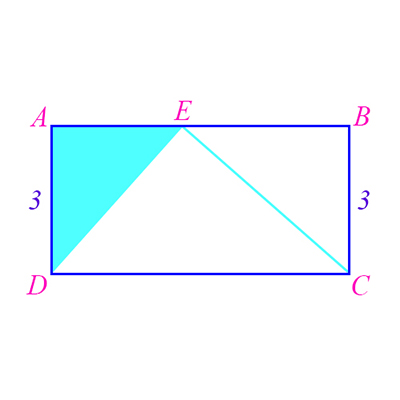In the diagram above, ABCD is a rectangle, with its shorter sides having length 3. What is the area of triangle AED?

(1) The perimeter of ABCD is 20.
(2) AE + EB = 7

Answer: E – Neither statement 1 nor statement 2 is sufficient, and together they are not sufficient.

Explanation:

Since we know the length of AD is 3, we just need to know the length of AE.(1) The perimeter of ABCD is 20.

Partial information. The perimeter is AB + BC + CD + DA. Since BC and DA each have length 3, then AB and CD must each have length 7. But this tells us nothing about the length of AE. Not sufficient.

(2) AE + EB = 7

Partial information. This tells us that AB has length 7, but it doesn’t tell us the length of AE. Not sufficient.

Alone, neither of these statements is sufficient, and together they are not sufficient, because they both tell us the same information – that AB has length 7. But they don’t tell us the length of AE.

Example 7)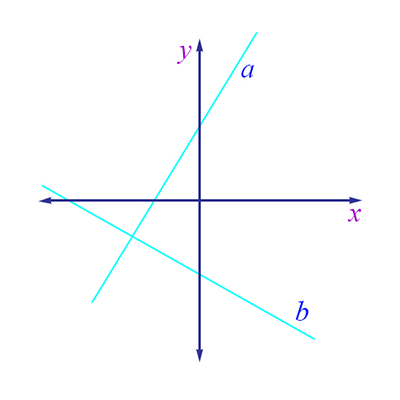In the diagram above, lines a and b are shown as intersecting lines on the normal xy-coordinate plane. Is line a perpendicular to line b?

(1) The slope of line a is 4.
(2) The equation of line b is x + 4y = -12.

Answer: C – Neither statement 1 nor statement 2 is sufficient, but together they are.

Explanation:

Two lines are perpendicular if the slope of one is the negative reciprocal of the other, or if they intersect at some point, and make complementary angles with the same line – for example, the x- or y-axis.(1) The slope of line a is 4.

Partial information. We need also the slope of line b. Not sufficient.

(2) The equation of line b is x + 4y = -12.

Partial information. From the equation of a line you can obtain the slope. In this case the slope of line b is -1/4. But we also need the slope of line a. Not sufficient.

Alone, neither of these statements is sufficient, but together they are sufficient, because from the equation of a line (statement 2) you can obtain the slope. In this case the slope of line b is – 1/4, which is the negative reciprocal of 4. Since the slope of a is 4 (statement 1), the two lines are perpendicular.

Example 8)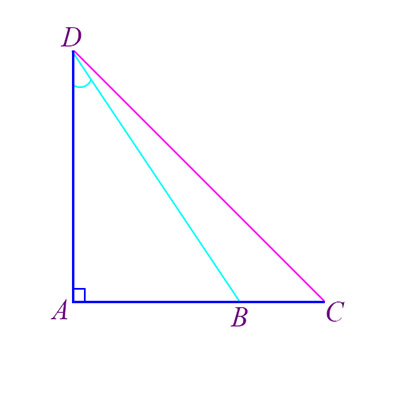In the figure above, ∠DAB is a right angle. What is the degree measure of ∠ADB?

(1) The degree measure of ∠DBC is 120.
(2) The sum of the degree measures of ∠BDC and ∠BCD is 60.

Answer: D – Both statement 1 and statement 2 are individually sufficient.

Explanation:(1) The degree measure of ∠DBC is 120.

Full information. ∠DBC is supplementary to ∠DBA, so we can calculate ∠DBA as \begin{align}60^\circ.\end{align} Using ∠DBA and the right angle, ∠DAB, and the fact that the angles in a triangle sum to 180, we can calculate ∠ADB as \begin{align}30^\circ.\end{align} Sufficient.

(2) The sum of the degree measures of ∠BDC and ∠BCD is 60.

Full information. If the sum of these two angles is 60, then the remaining angle in the triangle, ∠DBC, has degree measure 120. Since it is supplementary to ∠DBA, we can calculate ∠DBA as \begin{align}60^\circ.\end{align} Using ∠DBA and the right angle, ∠DAB, we can calculate ∠ADB as \begin{align}30^\circ.\end{align} Sufficient.